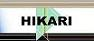| Home | Journals | Books | Paper submission | About us and our mission | News | Contact us |Site Search:

## Online Edition, Vol. 6, 2012, no. 45-48

M. A. Abdou, F. M. Al-Saedy
Generalized potential function in linear mixed integral equation
Int. Journal of Math. Analysis, Vol. 6, 2012, no. 45-48, 2199-2216.

E. Abu-Sirhan, O. H. H. Edely
On simultaneously remotal in L^1(\mu,X)
Int. Journal of Math. Analysis, Vol. 6, 2012, no. 45-48, 2217-2226.

Fan Aihua, Shen Yang, Zhang Huaiju
Limit theorems for delayed averages of random sequence
Int. Journal of Math. Analysis, Vol. 6, 2012, no. 45-48, 2227-2234.

F. M. Gaafar
Uniformly stable solution of a non-autonomous delayed system with Caputo fractional derivative
Int. Journal of Math. Analysis, Vol. 6, 2012, no. 45-48, 2235-2244.

Weakly sentinels for the distributed system with missing terms and with pollution in the boundary conditions
Int. Journal of Math. Analysis, Vol. 6, 2012, no. 45-48, 2245-2256.

E. Demir
On strictly transitive operator algebras
Int. Journal of Math. Analysis, Vol. 6, 2012, no. 45-48, 2257-2264.

Pankaj, S. S. Shukla, R. Prasad
Generalized CR-submanifolds of manifolds with a Sasakian 3-structure
Int. Journal of Math. Analysis, Vol. 6, 2012, no. 45-48, 2265-2275.

S. I. El-Ganaini
New soliton solutions of some important nonlinear systems via He's variational principle
Int. Journal of Math. Analysis, Vol. 6, 2012, no. 45-48, 2277-2286.

M. F. El-Sabbagh, S. I. El-Ganaini
New exact solutions of Broer-Kaup (BK) and Whitham Broer-Kaup (WBK) systems via the first integral method
Int. Journal of Math. Analysis, Vol. 6, 2012, no. 45-48, 2287-2298.

A. Sudhakaraiah, V. Raghava Lakshmi
Domination number of circular-arc overlap graphs: A new perspective
Int. Journal of Math. Analysis, Vol. 6, 2012, no. 45-48, 2299-2306.

D. Vidhya, R. Parimelazhagan
g*b-Continuous maps and pasting lemma in topological spaces
Int. Journal of Math. Analysis, Vol. 6, 2012, no. 45-48, 2307-2315.

S. Muthuvel, R. Parimelazhagan
b*-Closed sets in topological spaces
Int. Journal of Math. Analysis, Vol. 6, 2012, no. 45-48, 2317-2323.

A. Poongothai, R. Parimelazhagan
sb*-Closed sets in topological spaces
Int. Journal of Math. Analysis, Vol. 6, 2012, no. 45-48, 2325-2333.

V. Lampret
Estimating \pi easily and accurately
Int. Journal of Math. Analysis, Vol. 6, 2012, no. 45-48, 2335-2344.

A. Kaewcharoen
Common fixed points for four mappings in G-metric spaces
Int. Journal of Math. Analysis, Vol. 6, 2012, no. 45-48, 2345-2356.

R. Mathur
Integral representations of Exton's triple hypergeometric series
Int. Journal of Math. Analysis, Vol. 6, 2012, no. 45-48, 2357-2360.

L. Ciurdariu
On some Hermite-Hadamard type inequalities for functions whose power of the absolute value of derivatives are (\alpha,m)-convex
Int. Journal of Math. Analysis, Vol. 6, 2012, no. 45-48, 2361-2383.

M. Boulerhcha, Benbrik Abdelhaq, Lahrech Samir
Optimal bilinear control problems governed by evolution partial differential equation
Int. Journal of Math. Analysis, Vol. 6, 2012, no. 45-48, 2385-2395.

H. Mahzoon
New subclasses of analytic functions defined by subordination
Int. Journal of Math. Analysis, Vol. 6, 2012, no. 45-48, 2397-2403.

E. Movahednia, S. Eshtehar, Young Son
Stability of quadratic functional equations in fuzzy normed spaces
Int. Journal of Math. Analysis, Vol. 6, 2012, no. 45-48, 2405-2412.

A. Gorbenko, V. Popov
Usage of the Laplace transform as a basic algorithm of railroad tracks recognition
Int. Journal of Math. Analysis, Vol. 6, 2012, no. 45-48, 2413-2417.